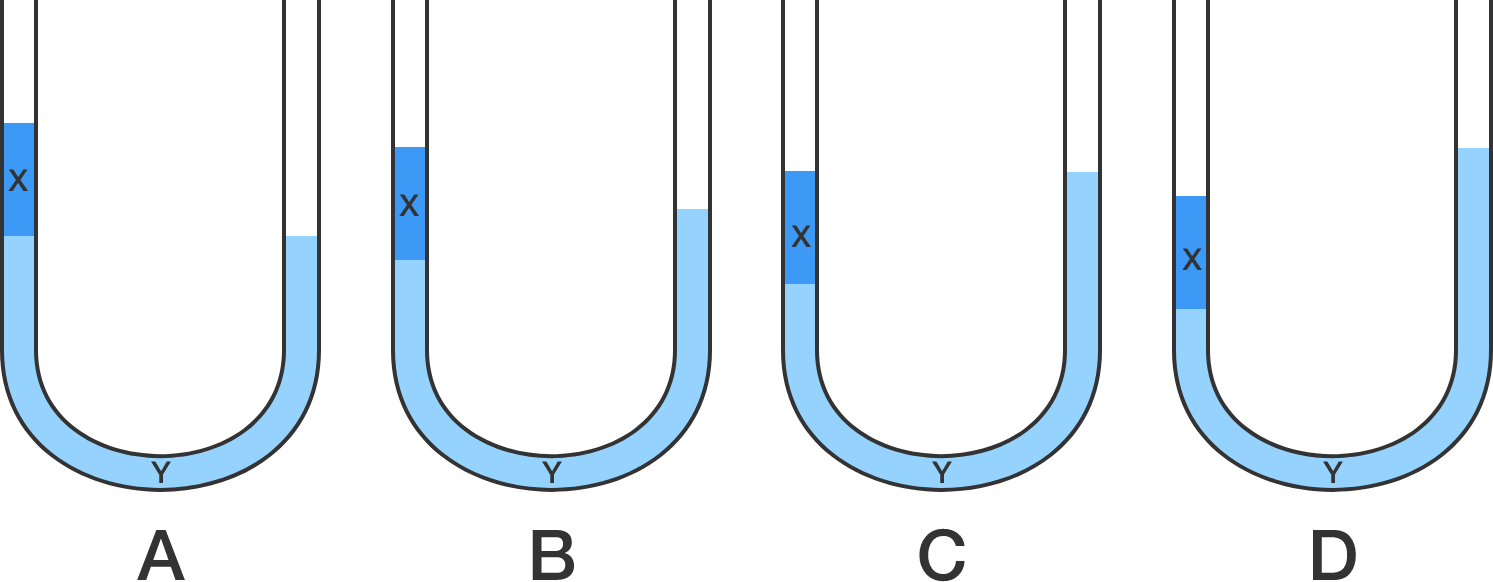# U-Tube!

Joe pours liquid $X$ (in dark blue) into the left arm of a U-tube that already contains liquid $Y$ (in light blue). The density of liquid $X$ is less than that of liquid $Y,$ and the two liquids are immiscible.

Which of the following represents the correct situation for the two liquids in the U-tube?×